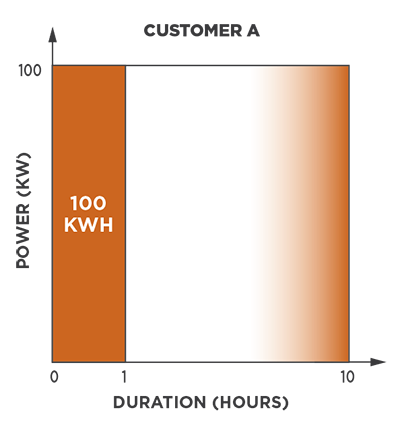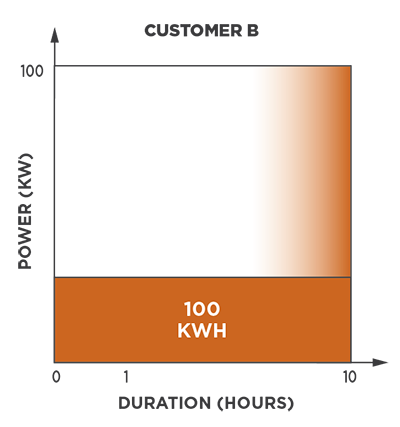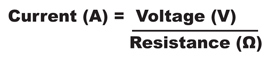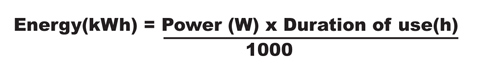# What is kWh, kW and kVA?

A kilowatt hour (kWh), a kilowatt (kW) and kilovolt ampere (kVA) are all units of measurement, but they serve different purposes. Understanding the difference between each can help you better manage your energy bill.

The primary difference between kWh, kW and kVA are in what they measure. A kWh is a measure of energy and kW is a measure of power. Power is the rate at which something uses energy, and energy is the capacity to do work. KVA is a measure of power, similar to KW but includes reactive power which is a factor for customers with considerable inductive loads.

We invoice most General Service and Industrial customers for power (demand) and energy (consumption) as two separate components. Demand charges are intended to recover the cost of facilities (such as power transformers, wires and power plants) available to provide the maximum amount of electricity which customer may require at any time.

Here's a quick comparison:

 Premise 1Premise 2Consider two premises:

Premise #1 has a total load of 100 kilowatts for 1 hour and consumed 100 kilowatt-hours. Premise #2 had a total load of 10 kilowatts for 10 hours and consumed 100 kilowatt-hours. Both premises had used the same amount of kilowatt-hours however NB Power must provide capacity of 100kw for premise #1 while premise #2 only needed 10kw of capacity.

## What is voltage?

Voltage is the difference in electrical potential between two points. It is expressed in volts (V) . (Note: The term potential also means voltage)

## What is current?

Current is the quantity of electrons flowing through a wire in one second. It is expressed in amperes (A) and is a function of voltage in volts (V) and resistance in ohms.## What is power?

Power is the rate at which the electricity is consumed. It is expressed in watts (W) or kilowatts (1 KW = 1000 W).The maximum power recorded during a certain period of time is called demand. It is measured in “KW” (Active Power) and /or “KVA” (Apparent Power).  NB Power uses 15-minute intervals for billing demand.

## What is energy?

Energy is the product of power supplied, multiplied by the length of time it is used. It is measured in kilowatt-hours (KWh).Usually used to express power in all forms, but is reserved to express real power. 1000 Watts (W) = 1 kiloWatt (kW).

Direct current flowing in a conductor is directly proportional to the potential difference between its ends. It is usually formulated as V = IR, where V is the potential difference, or voltage, I is the current, and R is the resistance of the conductor.

Resistance is a term that describes the forces that oppose the flow of electrons in a conductor.

The charges the electrical utility put on your bill for peak demand. The demand charges are usually derived from peak kW or kVA.